## Section4.3Velocity and Speed

### Subsection4.3.1Average Velocity

The ratio of displacement $\Delta \vec r$ to the interval $\Delta t$ over which displacement occured is called average velocity during that interval.

\begin{equation} \vec v_{\text{av}} = \dfrac{\Delta \vec r}{\Delta t} .\label{eq-average-velocity-vector-definition}\tag{4.3.1} \end{equation}

Since $\Delta \vec r$ is a vector and we are dividing it by a simple number, average velocity, $\vec v_{\text{av}}$ will also be a vector. Just as other vectors, we can represent velocity by an arrow of appropriate size and direction or analytically as components or in magnitude-direction form.

Noting that displacement $\Delta \vec r$ has the components $(\Delta x, \Delta y, \Delta z) \text{,}$ we can also cast Eq. (4.3.1) in the component form to obtain the following for the components of the average velocity.

\begin{equation*} v_{\text{av},x}= \dfrac{\Delta x}{\Delta t}, \ \ v_{\text{av},y}= \dfrac{\Delta y}{\Delta t}, \ \ v_{\text{av},z}= \dfrac{\Delta z}{\Delta t}. \end{equation*}

From the components, we can deduce the magnitude and direction as we would do for any other vector as illustrated in Checkpoint 4.3.1.

An ant is crawling randomly on the floor. At some time $t = 0 \text{,}$ the ant is at coordinates $(20\text{ cm}, -30\text{ cm})$ and some time later, at $t = 20\text{ sec} \text{,}$ it is at $(-10\text{ cm}, 10\text{ cm}) \text{.}$ What is the average velocity in (a) the component form and (b) in the magnitude-direction form?

Hint

First find the displacement vector in the component form.

(a) $(-1.5\text{ cm/s}, 2.0\text{ cm/s} ) \text{,}$ (b) $2.5\text{ cm/s},\ 53.1^{\circ}$ clockwise from negative $x$ axis.

Solution 1 (a)

(a) We first find the displacement vector in the component form.

\begin{equation*} \Delta x = -10-20 = -30\text{ cm},\ \ \Delta y = 10-(-30) = 40\text{ cm}. \end{equation*}

Therefore, the components of the average velocity are

\begin{align*} \amp v_{\text{av},x} = \dfrac{\Delta x}{\Delta t} = \dfrac{-30}{20} = -1.5\text{ cm/s}. \\ \amp v_{\text{av},y} = \dfrac{\Delta y}{\Delta t} = \dfrac{40}{20} = 2.0\text{ cm/s}. \end{align*}

Therefore, $\vec v_{\text{av}} = (-1.5\text{ cm/s}, 2.0\text{ cm/s} ) \text{.}$

Solution 2 (b)

(b) The magnitude and angle from components can be found from the same formulas that relate $(x, y)$ to $(r, \theta)\text{.}$

\begin{align*} \amp v_{\text{av}} = \sqrt{1.5^2 + 2^2} = 2.5\text{ cm/s}. \\ \amp \theta = \tan^{-1}\left( \dfrac{2}{-1.5}\right) = -53.1^{\circ}. \end{align*}

Now, we interpret the angle to get the direction. Note that the point $(-1.5, 2)$ is in the second quadrant. Therefore, the direction is in a clockwise angle of $53.1^{\circ}$ from the negative $x$ axis.

### Subsection4.3.2Average Speed

Average speed is different from average velociy, although we often tend to confuse them as same. For average speed, we divide the total distance traveled on the actual path by time taken, not just the direct distance between the initial point and the final point.

\begin{equation} \text{Average Speed } = \dfrac{\text{Distance Traveled}}{\text{Time Taken}}\tag{4.3.2} \end{equation}

The confusion comes from the fact that we use the same symbol for speed as we do for the magnitude of average velocity. To avoid the confusion, let us use symbol $v_{s,av}$ for the average speed, $d$ the distance traveled, and $t$ the time, then we have

\begin{equation} v_{s,av} = \dfrac{d}{t}\tag{4.3.3} \end{equation}

Average Speed is Different from Average Velocity: An extreme example that shows the difference between average speed and average velocity occurs in a cloed-loop motion. Let $T$ denote the time it takes to return after going around the closed path, say from your home to the grocery store and back to your home. Let $D$ be the total distance to the store and back. Average velocity here will be zero, since displacement is zero, but average speed will be $D/T\text{.}$

### Subsection4.3.3Instantaneous Velocity - Formal Definition

Average velocity and average speed do not tell us motion moment-by-moment. For that Isaac Newton, introduced the concept of instantaneous velocity based on concepts of limit and infinitesimal intervals.

If you find average velocity using Eq. (4.3.1) for different interval values, i.e., different values of $\Delta t\text{,}$ you will find that you get different velocities. Newton noticed that, as $\Delta t$ become increasingly small, the differences in average velocities become less and less in a well-defined way such that it is possible to define a unique velocity in the limit $\Delta t\rightarrow 0\text{.}$ Denoting the instantaneous velocity vector by $\vec v$ and the position vector as $\vec r$ we can write the definition of instantaneous velocity formally by

\begin{equation} \vec v = \lim_{\Delta t \rightarrow 0} \dfrac{\vec r\left(\text{at } (t+\Delta t)\right) - \vec r\left(\text{at } t\right)}{\Delta t} \equiv \frac{d\vec r}{dt}.\label{eq-instantaneous-velocity-3d-limit}\tag{4.3.4} \end{equation}

The magnitude of instantaneous velocity gives us the speed at that instant. Although, we noted above that average speed and magnitude of average velocity may be different, but instantaneous speed and magnitude of instantaneouos velocity are always same since averaging in Eq. (4.3.4) is over an infintesimal time interval.

Eq. (4.3.4) is an abstract definition, which we can make more accessibale by looking at the components. Denoting $\vec r\left(\text{at } (t+\Delta t)\right) - \vec r\left(\text{at } t\right)$ by $\Delta \vec r \equiv (\Delta x, \Delta y, \Delta z)\text{.}$

\begin{align*} \amp v_x= \lim_{\Delta t \rightarrow 0}\dfrac{\Delta x}{\Delta t} \equiv \frac{dx}{dt},\\ \amp v_y= \lim_{\Delta t \rightarrow 0}\dfrac{\Delta y}{\Delta t} \equiv \frac{dy}{dt},\\ \amp v_z= \lim_{\Delta t \rightarrow 0}\dfrac{\Delta z}{\Delta t} \equiv \frac{dz}{dt}. \end{align*}

These components of velocity are also called $x$-, $y$-, and $z$-velocity respectively. In a previous chapter, we have studied one-dimensional motion, where only one of them was non-zero. For general motion, you can expect all of them to be nonzero and you would need to keep track of them all.

Numbers $v_x\text{,}$ $v_y\text{,}$ and $v_z$ you would obtain this way are the Cartesian components of the vector $\vec v\text{.}$ Thus, once we have obtained $(v_x, v_y, v_z)\text{,}$ we can deduce the magnitude and direction of the velocity vector as we do for any vector. For instane, magnitude of velocity, i.e., speed, will simply be the magnitude of the vector.

\begin{equation} v = \sqrt{v_x^2 + v_y^2 + v_z^2}.\tag{4.3.5} \end{equation}

For direction of $\vec v\text{,}$ we will use either polar angle in case of a motion in a plane, or spherical cordinate angles in case of motion in a three-dimensional space.

### Subsection4.3.4Velocity from Slopes of $(x, y, z)$ vs $t$ Plots.

When we studied one dimensional motion on $x$ axis, we noted that $v_x$ was the derivative of $x$ with respect to $t\text{.}$ The value of derivative at a point is equal to the slope of the tangent to the function at that point. Therefore, we were able to compute $v_x$ by a plot of $x$ versus $t\text{.}$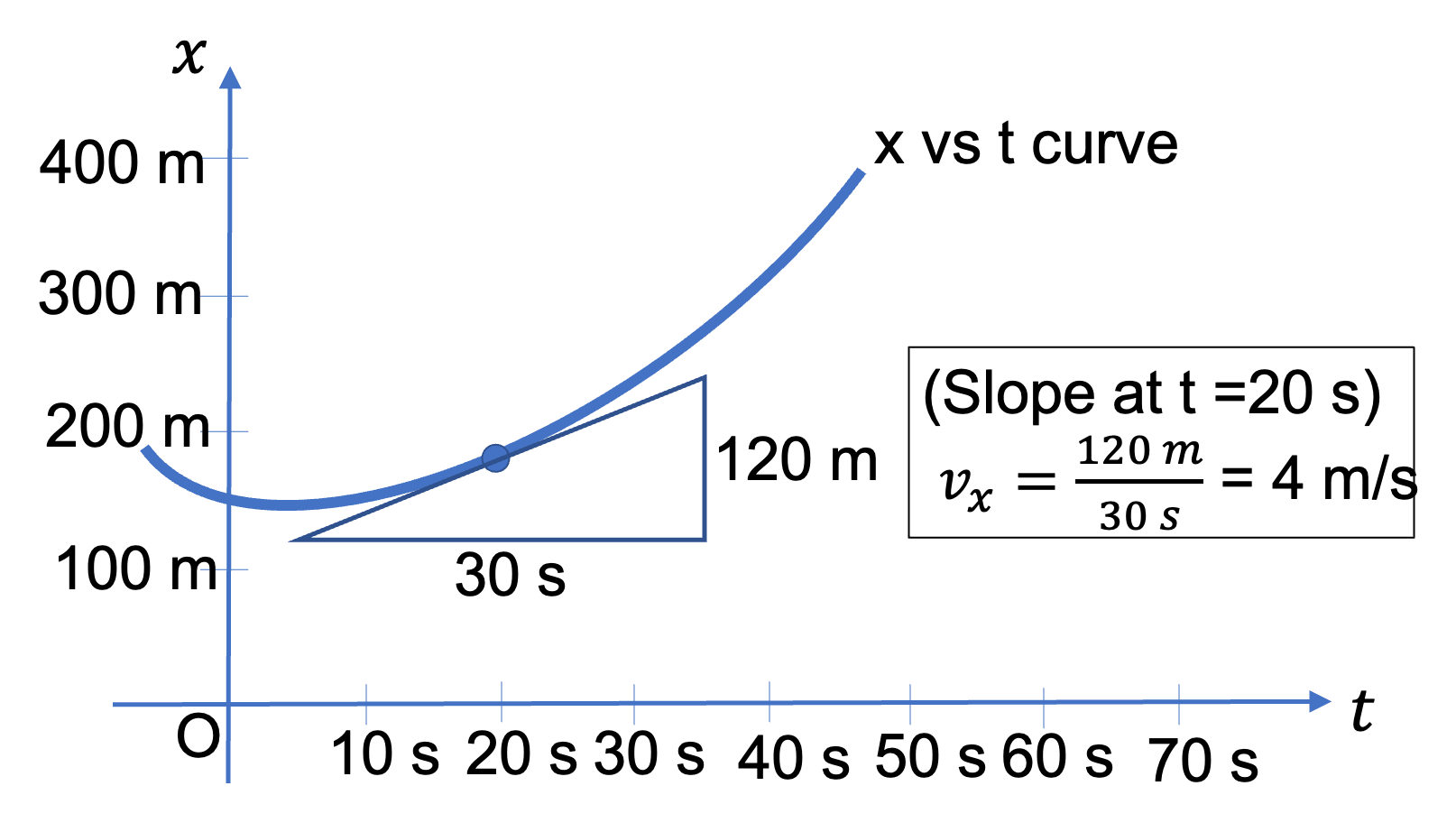Figure 4.3.2. $v_x$ from slope.

More generally, velocity (vector) is derivative $\vec r$ as defined in Eq. (4.3.4). We cannot plot $\vec r$ versus $t$ like a function, since a vector has both quantitative (the magnitude) and qualitative properties (the direction). But, we can think of $\vec r$ as a composite of three functions, $x(t)\text{,}$ $y(t)\text{,}$ and $z(t)\text{,}$ where I have placed $t$ in the argument to emphasize the independent variable being $t$ here. Plots of $x(t)\text{,}$ $y(t)\text{,}$ and $z(t)$ versus $t$ allows us to find $v_x\text{,}$ $v_y\text{,}$ and $v_z\text{,}$ respectively.

\begin{align*} \amp v_x= \text{Slope of tangent to } x \text{ versus } t \text{ plot}.\\ \amp v_y= \text{Slope of tangent to } y \text{ versus } t \text{ plot}.\\ \amp v_z = \text{Slope of tangent to } z \text{ versus } t \text{ plot}. \end{align*}

Once we have obtained $(v_x,\ v_y,\ v_z)\text{,}$ we can deduce the magnitude and direction of the velocity vector as usual.

The figure below shows an example of calculation of the $x$ component of instantaneous velocity from a plot of $x$ vs $t\text{.}$ To obtain $v_x$ at an instant, say at $t=t_1\text{,}$ we draw a tangent to the curve at $t=t_1$ as shown in the figure.

The rise of the tangent line gives the change in position $\Delta x$ along the axis and the run of the tangent gives the duration $\Delta t$ for that change. Therefore, slope of the tangent line, obtained by dividing $\Delta x$ by $\Delta t$ gives the rate at which $x$ coodinate changes at $t_1\text{,}$ i.e, the $v_x$ at $t_1\text{.}$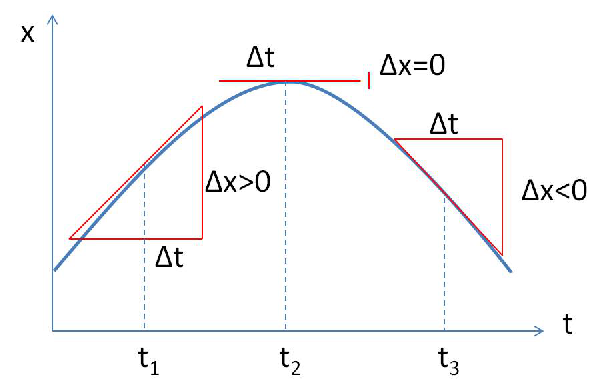At time $t_1\text{,}$ the slope of the tangent, i.e., $v_x\text{,}$ is positive, meaning the velocity is pointed in the positive $x$ direction for a one-dimensional motion on the $x$ axis.

Just to remind you that velocity for one-dimensional motion on the $x$ axis is $\vec v = v_x \hat i\text{,}$ where $\hat i$ is the unit vector pointed towards the positive $x$ axis. Note that at time $t_2$ in the figure, the slope is zero, meaning that the $v_x$ is zero there. At time $t_3\text{,}$ the slope is negative, meaning the velocity will be pointed in the negative $x$ axis direction for a one-dimensional motion.

I wish to emphasize here that an $x$ vs $t$ plot gives you only $v_x \text{.}$ In a three-dimensional motion, you will need $x$ vs $t$ , $y$ vs $t \text{,}$ and $z$ vs $t$ so that you can deduce $v_x \text{,}$ $v_y \text{,}$ and $v_z$ of velocity. You can then construct the magnitude and direction of the velocity vector from them.

Google maps shows that a road trip from Boston to Los Angeles will cover $4800 \text{ km}$ and take $48 \text{ hr}\text{.}$ If you were to dig a direct tunnel through the Earth between Boston and Los Angeles, you would find that the direct distance is approximately $4100 \text{ km}\text{.}$

(a) What is the average speed for the trip in km/h? (b) What is the average velocity for the trip?

Hint

(a) $v = d/t\text{,}$ (b) $\vec v_\text{av} = \Delta\vec r /\Delta t\text{.}$

(a) $100\text{ km/hr}\text{,}$ (b) $84\text{ km/h}$ in the direction in tunnel from Boston to Los Angeles.

Solution 1 (a)

(a) To obtain average speed, we divide the actual distance, as given by the road trip distance, by time.

\begin{equation*} v_s = \dfrac{4800 \text{ km}}{48 \text{ hr}} = 100\text{ km/hr}. \end{equation*}
Solution 2 (b)

(b) Average velocity $(\vec v_{\text{ave}})$ is a vector - so, we need to specify both the magnitude and direction.

The direction here is simple to state: it is along the direct line from Boston towards Los Angeles, as in the tunnel.

The magnitude of the average velocity will be equal to the direct distance, not the distance on the road trip, divided by time.

\begin{equation*} v_{\text{ave}} = \dfrac{4100\text{ km}}{48\text{ hr}} = 84\text{ km/h}. \end{equation*}

Suppose a cannon ball is fired with a speed $50 \text{ m/s}$ at an angle $40^{\circ}$ with the horizontal direction. A coordinate system is chosen such that the $x$ axis points horizontally and the positive $y$ axis points vertically up. What are the $x$ and $y$ components of the velocity vector?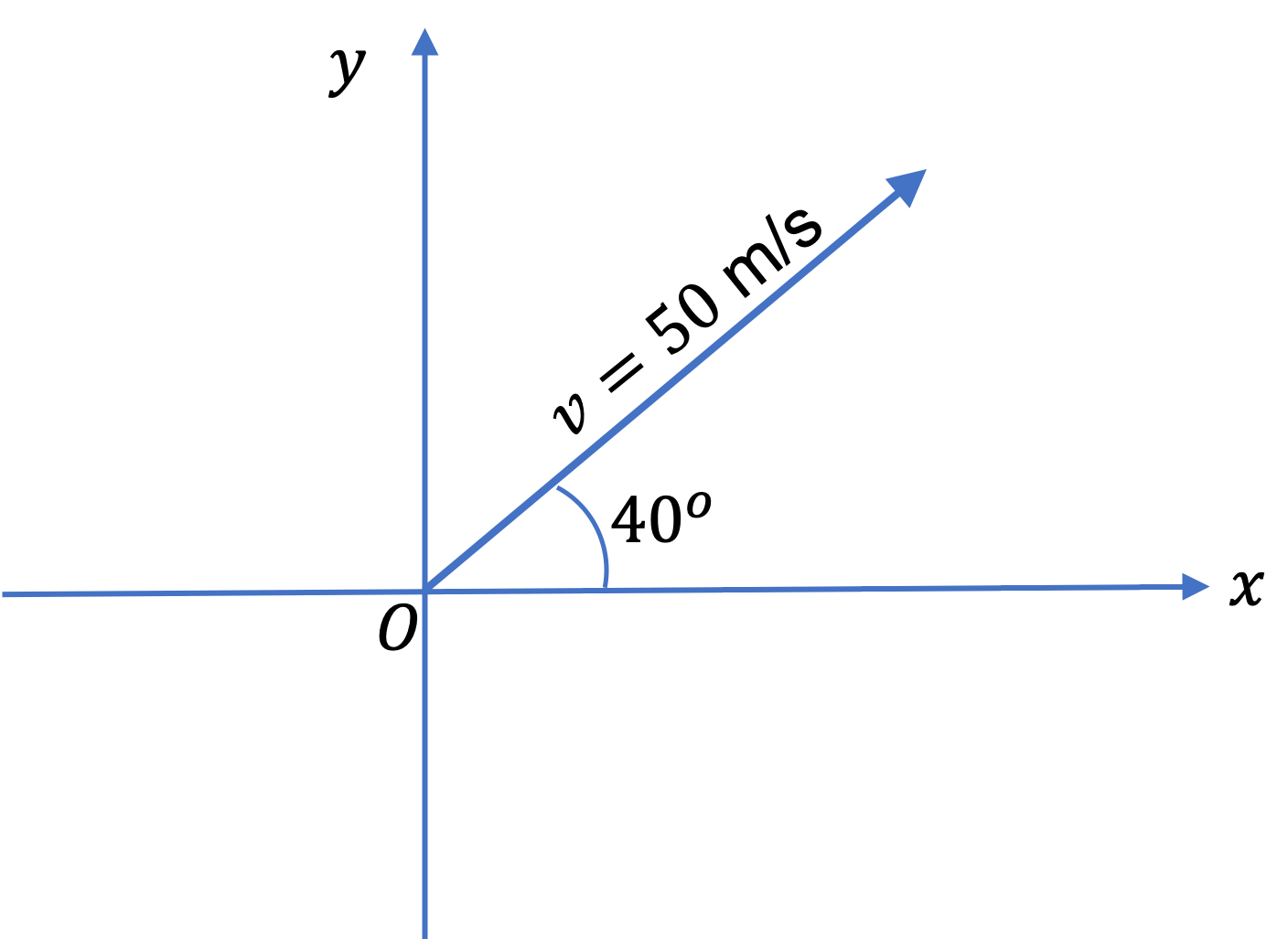Hint

Project the vector on $x$ axis to get $v_x\text{.}$ similarly for $v_y\text{.}$

$v_x = 38\text{ m/s} \text{,}$ $v_y = 32\text{ m/s} \text{.}$

Solution

Project a vertical line from the tip of the arrow to the $x$ axis (with the tail of the arrow at the origin. The length on the $x$ axis from origin is the $v_x \text{.}$ Trigonometry tells us that this should be hypotehnuse (which equal to the speed $v$) times cosine.

\begin{equation*} v_x = v\, \cos\, \theta = 50\text{ m/s}\, \cos\, 40^{\circ} = 38\text{ m/s}. \end{equation*}

Similiarly, the projection on the $y$ axis gives $v_y = v\, \sin\, \theta\text{,}$ which evaluates to $32\text{ m/s} \text{.}$

A cannon ball is fired at an angle of $30^{\circ}$ above the horizon at a speed of $40\text{ m/s}\text{.}$ Consider positive $x$ axis horizontally in the general direction the ball will fly and positive $y$ axis vertically up. (a) Draw the vector in the $xy$ plane and from its projections on the axes, identify the components on the diagram. (b) What are the $x$ and $y$ components of the velocity vector?

Hint

Project the vector on $x$ axis to get $v_x\text{.}$ similarly for $v_y\text{.}$

(b) $34.64\text{ m/s}, 20\text{ m/s}\text{.}$

Solution 1 (a)

(a) Lets draw the vector first and identify the $x$ and $y$ components in the diagram so that we can identify the trig we need. For clarity I will show only the $x$ component.

The diagram illustrates the projection on the $x$ axis to get the $x$ component $v_x\text{.}$ We draw the velocity vector with tail at the origin, and then we drop a perpendicular from the tip to each axis - figure shows only to $x$ axis for clarity. You should do similarly for the $y$ component.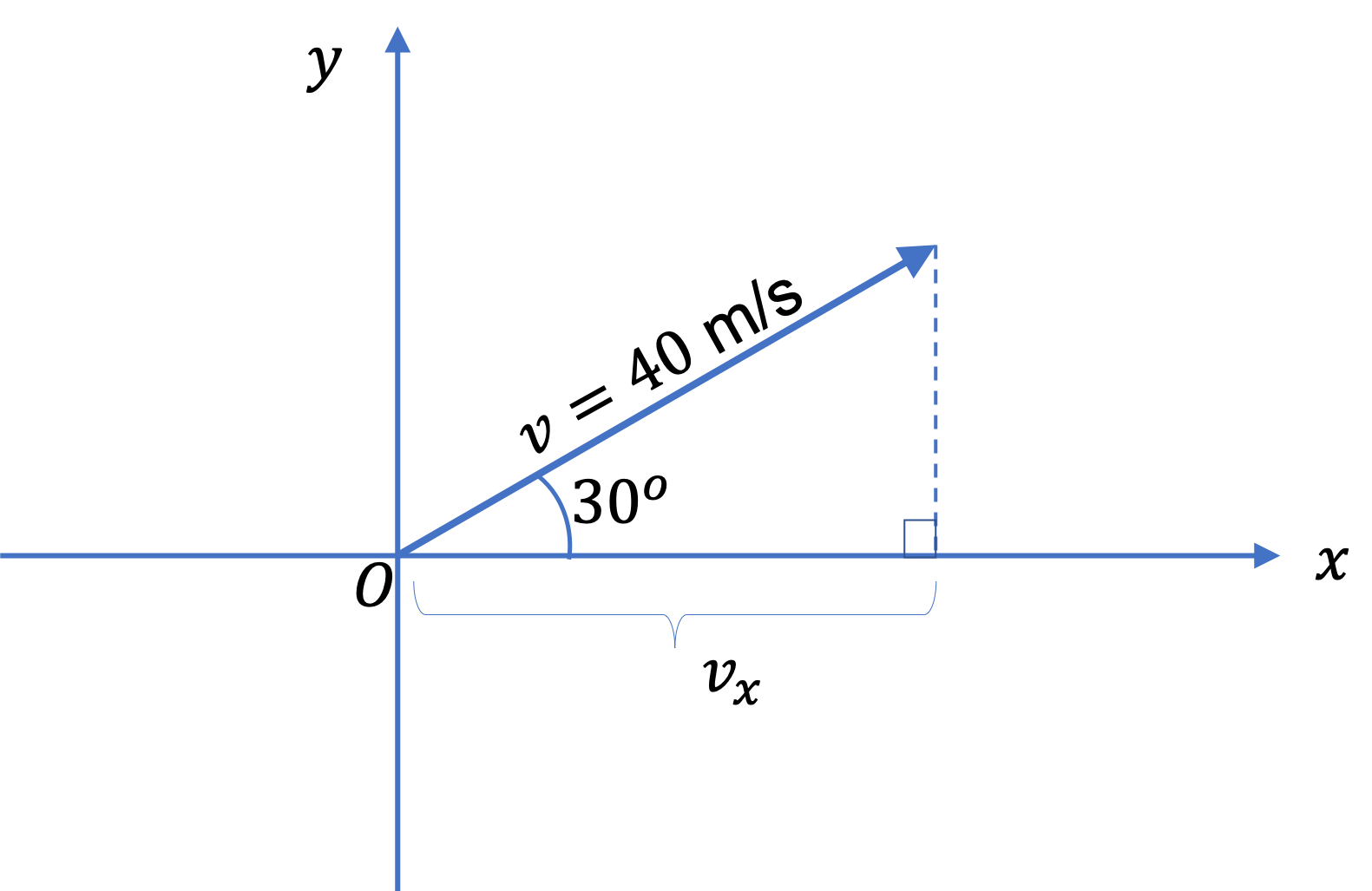Solution 2 (b)

(b) We are going to omit units in the calculations, but put back the units in the answers. From the figure it is clear that

\begin{align*} v_x \amp = v \cos\theta = 40 \times \cos\, 30^{\circ} = 34.64\text{ m/s}. \end{align*}

Similarly,

\begin{align*} v_y\amp = v \sin\theta = 40 \times \sin\, 30^{\circ} = 20\text{ m/s}. \end{align*}

Suppose a football is on a downward path. The $x$ and $y$ components of its velocity are $(-30\text{ m/s}, -40\text{ m/s}) \text{.}$ Find its magnitude and direction. Assume positive $x$ axis points East and positive $y$ axis points Up.

Hint

Use formulas for $(r, \theta)$ in terms of $(x,y) \text{.}$

$50\text{ m/s} \text{,}$ $53.1^{\circ}$ South of West.

Solution

The magnitude and angle of any vector are related to the components by the same formulas as $(r, \theta)$ for position vector are to $(x,y) \text{.}$ Therefore,

\begin{align*} \amp v = \sqrt{v_x^2 + v_y^2} = 50\text{ m/s}, \\ \amp \theta = \tan^{-1}\left(\dfrac{v_y}{v_x} \right) = 53.1^{\circ}. \end{align*}

We now need to interpret this angle to get the direction. The point $(-30, -40)$ is in the third quadrant, therefore, the direction is counterclockwise from the negative $x$ axis. This gives the directioj to be $53.1^{\circ}$ South of West. as shown in Figure 4.3.8.

A ball is rolling on the floor. The position of the ball is recorded as $(x,y)$ of a coordinate system. The $z$ coordinate of the ball is always zero in this coordinate system and therefore ignored. The plots of $x$ vs $t$ and $y$ vs $t$ are displayed here. Find the velocity of the ball at (a) $t = 3\text{ sec}\text{,}$ (b) $t=5\text{ sec}\text{,}$ and (c) $t=7\text{ sec}\text{.}$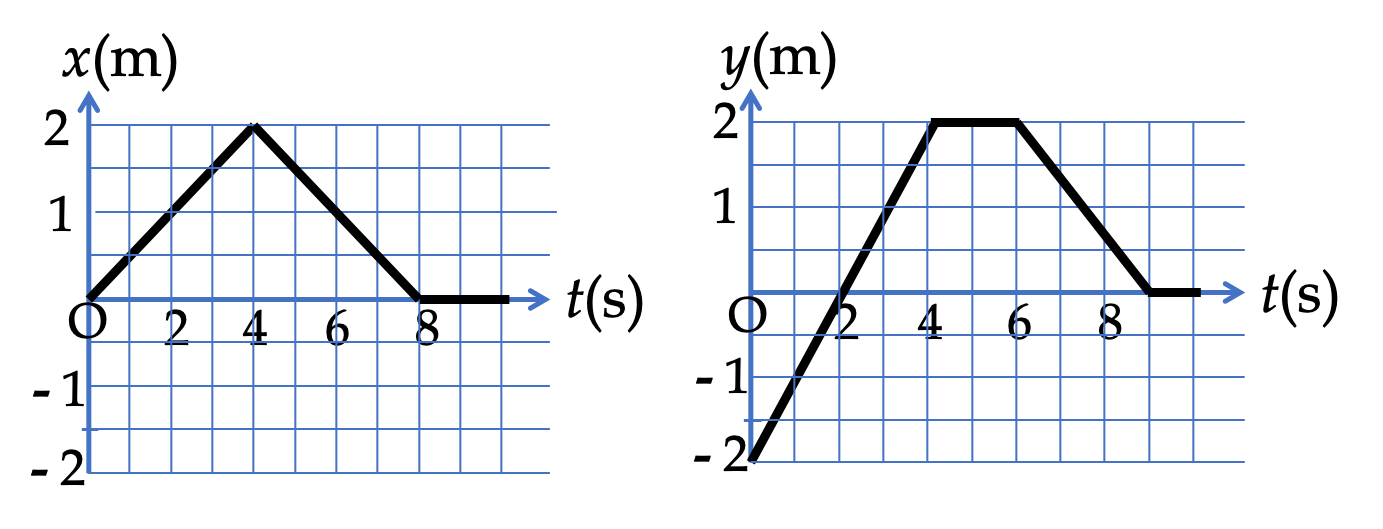Hint

(a) Find $v_x$ and $v_y$ from slopes at $t = 3\text{ sec}\text{.}$

(a) $(0.5\ \text{ m/s},\ 1.0\text{ m/s} )\text{,}$ (b) $(-0.5\text{ m/s},\ 0) \text{,}$ (c) $(-0.5\text{ m/s},\ -1.0\text{ m/s})\text{.}$

Solution 1 (a)

(a) The slope of the $x$ vs $t$ grasph at $t = 3$ is obtained by two points on the line through the $(t=3, x=1.5)$ point on the graph. Lets pick the second point on this line to be $(t=0, x=0)\text{.}$ Then we get

\begin{equation*} v_x = \dfrac{1.5-0}{3-0} \text{ m/s} = \dfrac{1}{2} \text{ m/s}. \end{equation*}

Similarly we pick two points on the line through $(t=3, y=1.0)$ point. Let the other point on this line be $(t=2, y=0)\text{.}$ This gives

\begin{equation*} v_y = \dfrac{1.0-0}{3-2} \text{ m/s} = 1.0 \text{ m/s}. \end{equation*}

Therefore, the velocity in component form in the $xy$-plane is $(0.5\ \text{ m/s}\text{,}$ $1.0\text{ m/s} )\text{.}$

Solution 2 (b)

(b) Similary, we get $v_x = -0.5\text{ m/s}$ and $v_y = 0\text{.}$

Solution 3 (c)

(c) I got $v_x = -0.5\text{ m/s}$ and $v_y = -1.0\text{ m/s}\text{.}$

### Subsection4.3.5Direction of Instantaneous Velocity from Trajectory

If you can draw the path of the moving object in space, you will get some curvy trajectory in space. The direction of velocity at some instant, then is the direction of the tangent to this trajectory as shown in Figure 4.3.10.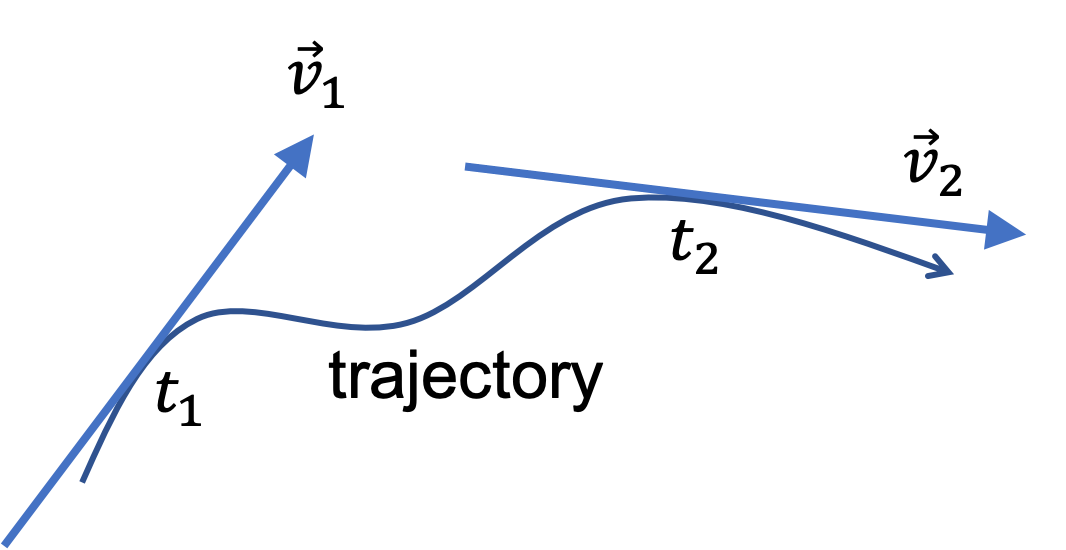Figure 4.3.10. The direction of velocity vector can be deduced from tangent to the path/trajectory of the object in space. The figure shows directions of velocity vectors at instants $t_1$ and $t_2\text{.}$

The trajectory of a projectile is shown in the figure below with the assumption that the initial velocity was $80\text{ m/s}$ at the angle of $72^{\circ}$ from the horizontal direction. The position of the projectile at successive $1 \text{ sec}$ instants are shown in the figure

You can see that the direction as well as the magnitude of the velocity of the projectile changes with time. As the projectile rises, the distance covered in each subsequent second decreases as a result of slowing down. At the top of the trajectory, the projectile only has horizontal velocity since the vertical component of the velocity is zero there. The velocity is never pointed vertically straight down since the projectile always has a non-zero horizontal component of the velocity.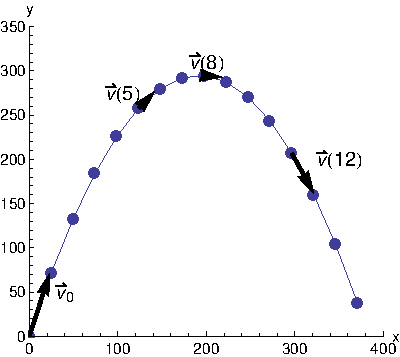Consider a child going in a circular path in a merry-go-round at a constant speed of $5 \text{ m/s}\text{.}$ Draw a circle and show velocity vectors at four different points of the circle.

Hint

Direction is tangent to the circle.

see solution

Solution

At each point, the velocity is tangent to the trajectory as shown in Figure 4.3.13.

### Subsection4.3.6(Calculus) Instantaneous Velocity and Instantanous Speed

To study accelerating motion, we need to understand not just average velocity, but also velocity throughout the motion. The instantaneous velocity and instantanous speed are mathematical concepts that tell us how fast the object is moving at an instant. Additionally, the instantaneous velocity also tells us which way the object is headed next.

We need Calculus to define these quantities - so if you have not had Calculus yet, you can skip this section - just note that the magnitude of the velocity at an instant says how fast it is moving at that instant, which is same as speed at that instant, and the direction of the velocity vector tells us the direction in which the object is headed next.

Velocity at an instant, called the instantaneous velocity or simply as velocity, is defined as the average velocity over infinitesimal interval around the instant of interest. This requires taking a limit of the average velocity as we decrease the infinitesimal inerval. This results in the velocity equal to the derivative of position vector $\vec r\text{.}$

\begin{equation} \vec v = \dfrac{d\vec r}{dt}.\tag{4.3.6} \end{equation}

One way to think about derivative of a vector is to write it in Cartesian components. Since $\vec r = x \hat i + y \hat j + z \hat k\text{,}$ the Cartesian components of velocity vector can be obtained by taking derivatives of position coordinates.

\begin{equation} \vec v = \left( \dfrac{dx}{dt}, \dfrac{dy}{dt}, \dfrac{dy}{dt} \right),\tag{4.3.7} \end{equation}

Let us denote, as usual, the Catesian components of $\vec v$ by $v_x \text{,}$ $v_y \text{,}$ and $v_z$ . Then, we will have each component of the velocity vector equal to just a derivative of the corresponding coodinate.

\begin{equation} v_x = \dfrac{dx}{dt},\ \ v_y = \dfrac{dy}{dt},\ \ v_z = \dfrac{dz}{dt}.\tag{4.3.8} \end{equation}

Magnitude of Instantaneous Velocity. The magnitude of the velocity vector tells us how fast the object is moving. This is what we also mean by speed. Therefore, the magnitude of instantaneous velocity vector is the same thing as instantaneous speed. From the Cartesian components of the velocity, we can immediatrely write a formula for speed in terms of the derivatives of the coordinates.

\begin{align} \text{Speed, } v \amp = \sqrt{v_x^2 + v_y^2 + v_z^2} \tag{4.3.9}\\ \amp = \sqrt{ \left( \dfrac{dx}{dt} \right)^2 + \left( \dfrac{dy}{dt} \right)^2 + \left( \dfrac{dz}{dt} \right)^2} \tag{4.3.10} \end{align}

Direction of Instantaneous Velocity. The above procedure provides a way of finding the components of the instantaneous velocity,, viz., $\vec v = (v_x, v_y, v_z) \text{.}$ From components $(v_x, v_y, v_z) \text{,}$ we can also get the direction of $\vec v$ in space as we would for any vector quantity. For instance, if the velocity vector was in the $xy$-plane, the $z$ component will be zero, and we would write $\vec v = (v_x, v_y) \text{.}$ Then, the angle with respect to the $x$ axis will be

\begin{equation*} \theta = \tan^{-1}\left( {\dfrac{v_y}{v_x}}\right). \end{equation*}

In case of a fully three-dimensional situation, we would use the polar and azimuthal angles, as usual, to indicate the direction.

The position of a pendulum bob is given by $\vec r = 5 \cos(t)\, \hat i + 5\sin(t)\, \hat j$ in units of m with units of second for $t \text{.}$ Find the velocity of the bob at $t = 2 \text{ s}\text{.}$

Hint

Take derivatives and evaluate at $t=2 \text{ s}\text{.}$

$v_x = -4.55 \text{ m/s, } v_y = -2.08 \text{ m/s}.$ or $5.0\text{ m/s},\ 24^{\circ}$ counterclockwise from the negative $x$ axis.

Solution

Note that the position vector has given us the $x(t)$ and $y(t) \text{,}$ the coordinates of the pendulum bob as functions of time $t \text{.}$ From these we can immediately obtain the $v_x$ amnd $v_y \text{,}$ the components of the velocity vector.

\begin{align*} \amp v_x = \dfrac{dx}{dt} = \dfrac{d}{dt}\left( 5\, \cos\,t\right) = -5\,\sin\,t\\ \amp v_y = \dfrac{dy}{dt} = \dfrac{d}{dt}\left( 5\, \sin\,t\right) = 5\, \cos\,t \end{align*}

Evaluating these for $t = 2$ gives the following once we put the units back in.

\begin{equation*} v_x = -4.55 \text{ m/s, } v_y = -2.08 \text{ m/s}. \end{equation*}

From this we get the magnitude of velocity to be $5.0\text{ m/s}$ and angle with the $x$ axis to be

\begin{equation*} \theta = \tan^{-1}\left( 2.08/4.55\right)= 24^{\circ}. \end{equation*}

Since the point $(-4.55, -2.08)$ is in the third quadrant, the direction of the velocity is $24^{\circ}$ counterclockwise from the negative $x$ axis.